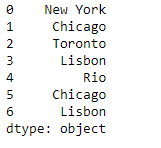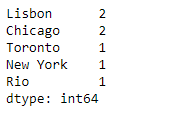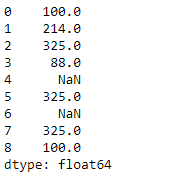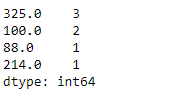# Python | Pandas Series.value_counts()

Python is a great language for doing data analysis, primarily because of the fantastic ecosystem of data-centric python packages. Pandas is one of those packages and makes importing and analyzing data much easier.

Pandas series is a One-dimensional ndarray with axis labels. The labels need not be unique but must be a hashable type. The object supports both integer- and label-based indexing and provides a host of methods for performing operations involving the index.

Pandas` Series.value_counts()` function return a Series containing counts of unique values. The resulting object will be in descending order so that the first element is the most frequently-occurring element. Excludes NA values by default.

Syntax: Series.value_counts(normalize=False, sort=True, ascending=False, bins=None, dropna=True)

Parameter :
normalize : If True then the object returned will contain the relative frequencies of the unique values.
sort : Sort by values.
ascending : Sort in ascending order.
bins : Rather than count values, group them into half-open bins, a convenience for pd.cut, only works with numeric data.
dropna : Don’t include counts of NaN.

Returns : counts : Series

Example #1: Use `Series.value_counts()` function to find the unique value counts of each element in the given Series object.

 `# importing pandas as pd ` `import` `pandas as pd ` ` `  `# Creating the Series ` `sr ``=` `pd.Series([``'New York'``, ``'Chicago'``, ``'Toronto'``, ``'Lisbon'``, ``'Rio'``, ``'Chicago'``, ``'Lisbon'``]) ` ` `  `# Print the series ` `print``(sr) `

Output :Now we will use `Series.value_counts()` function to find the values counts of each unique value in the given Series object.

 `# find the value counts ` `sr.value_counts() `

Output :As we can see in the output, the `Series.value_counts()` function has returned the value counts of each unique value in the given Series object.

Example #2: Use `Series.value_counts()` function to find the unique value counts of each element in the given Series object.

 `# importing pandas as pd ` `import` `pandas as pd ` ` `  `# Creating the Series ` `sr ``=` `pd.Series([``100``, ``214``, ``325``, ``88``, ``None``, ``325``, ``None``, ``325``, ``100``]) ` ` `  `# Print the series ` `print``(sr) `

Output :Now we will use `Series.value_counts()` function to find the values counts of each unique value in the given Series object.

 `# find the value counts ` `sr.value_counts() `

Output :As we can see in the output, the `Series.value_counts()` function has returned the value counts of each unique value in the given Series object.

Whether you're preparing for your first job interview or aiming to upskill in this ever-evolving tech landscape, GeeksforGeeks Courses are your key to success. We provide top-quality content at affordable prices, all geared towards accelerating your growth in a time-bound manner. Join the millions we've already empowered, and we're here to do the same for you. Don't miss out - check it out now!

Previous
Next